# Non Verbal Reasoning - Image Analysis

### Exercise :: Image Analysis - Section 2

1.

Select the alternative in which the specified components of the key figure (X) are found.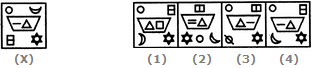A. 1 B. 2 C. 3 D. 4

Explanation:

No answer description available for this question. Let us discuss.

2.

Select the alternative in which the specified components of the key figure (X) are found.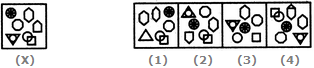A. 1 B. 2 C. 3 D. 4

Explanation:

No answer description available for this question. Let us discuss.

3.

Select the alternative in which the specified components of the key figure (X) are found.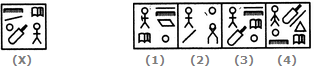A. 1 B. 2 C. 3 D. 4

Explanation:

No answer description available for this question. Let us discuss.

4.

Select the alternative in which the specified components of the key figure (X) are found.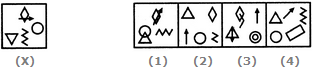A. 1 B. 2 C. 3 D. 4

Explanation:

No answer description available for this question. Let us discuss.

5.

Select the alternative in which the specified components of the key figure (X) are found.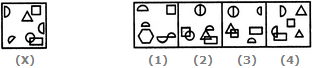A. 1 B. 2 C. 3 D. 4